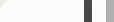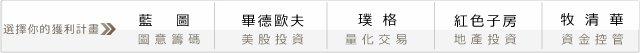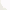## 累計損益的回檔，DD怎麼用R寫?

profit = sample(-20:25, 200, replace=TRUE)    ##隨機產生損益向量

DD = rep(0, length(profit))     ##產生向量，記錄目前DD
topprofit = rep(profit, length(profit))    ##紀錄過去的最高累計獲利

for (m in 2:length(profit)){    ##開始計算損益向量裡的回檔
if (sum(profit[1:m]) > topprofit[m-1]){
##目前累計獲利 > 過去最高獲利 i.e.創新高

topprofit[m:length(profit)]=sum(profit[1:m])
## 更新創新高的值到 topprofit

}
DD[m]=sum(profit[1:m])-topprofit[m]
## DD的定義: 目前累計獲利 - 歷史新高
}

ts.plot(cbind(cumsum(profit),DD),col=c("red","blue"))  ##畫圖

for (m in 2:length(profit)){
if (topprofit[m]>topprofit[m-1]){
points(m,topprofit[m],pch=4,col="purple")
}     ##當獲利創新高，打個紫色XX
}

abline(h=0,col="green") ##畫水平線

(ps: 由於每個人的損益向量是隨機產生，所以每個人的圖都不一樣)

#### 0 意見: